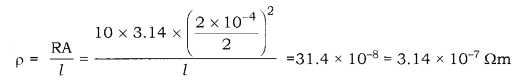# A wire is 1.0m long 0.2mm in diameter and has a resistance of 10Ω. Calculate the resistivity of its material

A wire is 1.0m long 0.2mm in diameter and has a resistance of 10Ω. Calculate the resistivity of its material.

We have,d = Diameter of the wire = 0.2mm = 2 × 10-4 m
l = Length of the wire = 1 m
r = 10Ω
p = Resistivity of the wire.Therefore, the resistivity of the material is 3.14 × 10-7 Ωm.